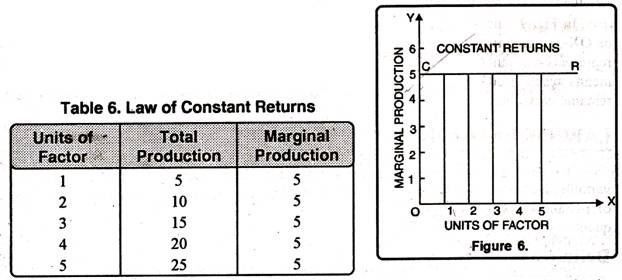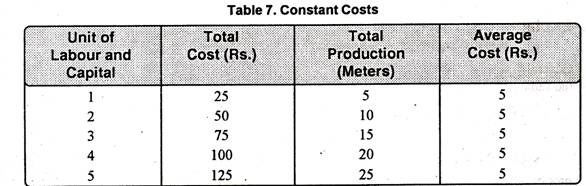# Law of Constant Marginal Returns

Law of Constant Marginal Returns or Law of Constant Costs!

The law of constant returns is also empirically valid and operates in the transitional stage between the Law of Diminishing Returns as well as The Law of Increasing Returns.

According to the Law, when in order to increase output, units of labour and capital are increased; output and cost also increase in the same proportion.

### Definitions:

“If the actions of law of increasing and diminishing returns are balanced we have the law of constant returns.” Marshall

“The law of constant returns states that when all the productive services are increased in a given proportion, the product is increased in the same proportion.” Stigler

### Explanation of the Law:

The law of constant returns can be explained in the forms:

(A) Law of Constant Returns and

(B) Law of Constant Costs.

#### (A) Law of Constant Returns:

The law of constant returns can be illustrated with the help of Table 6. This table indicates that production will be made by using different units of labour and capital.Table 6 reveals that production increases in the same ratio in which the factors of production are increased. When the factors of production are doubled, production is doubled from 5 meters to 10 meters. Likewise, it increases to 10, 15, 20, and 25 respectively. In this stage marginal and average production remains constant.

As seen in figure 6 factors of production and marginal production are represented along OX-axis and OY-axis respectively. Curve CR is parallel to OX.

This shows that with the increase in the factors of production, marginal production increases in the same quantity that is 5 per unit. It means that production is increasing in the same ratio in which factors of production are increasing.

#### (B) Law of Constant Costs:

Law of Constant Returns can also be explained in term of costs. According to it average and marginal cost of production remain constant as a result of additional application of the variable factor along with the fixed factor of production. It means, whether production increases or decreases, no change occurs in average cost. The law of constant cost can be elaborated with the help of table 7 and Figure 7 below.Table 7 shows that total production and total cost increase in the same proportion. As a result, there is no change in average cost due to increase in production. Average cost remains Rs. 5.00 per unit. In Fig. 7 units of labour and capital are given on OX-axis and average cost on OY-axis. CC curve represents constant cost. It is parallel to OX-axis. It means when production increases, average cost remains constant.